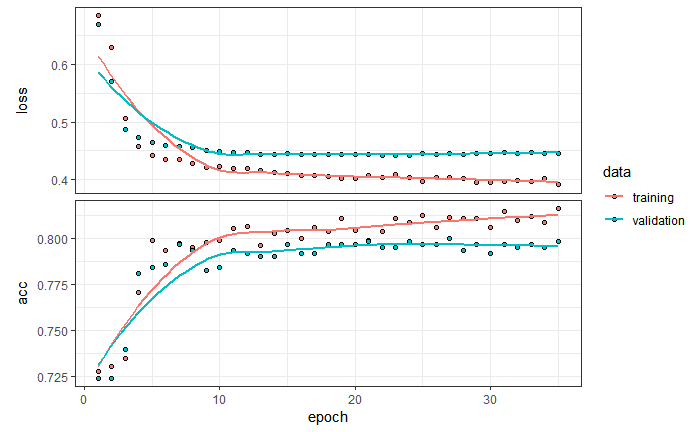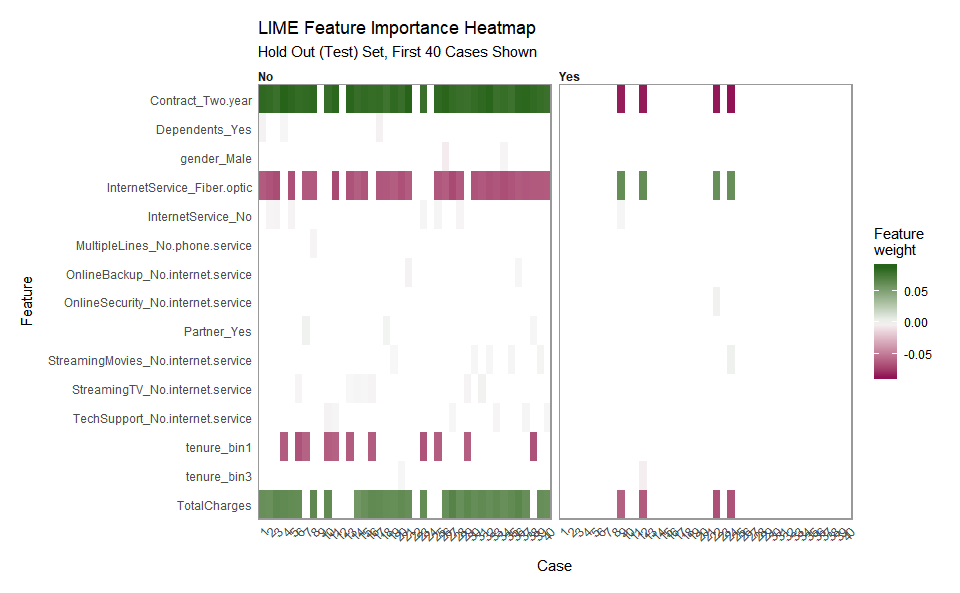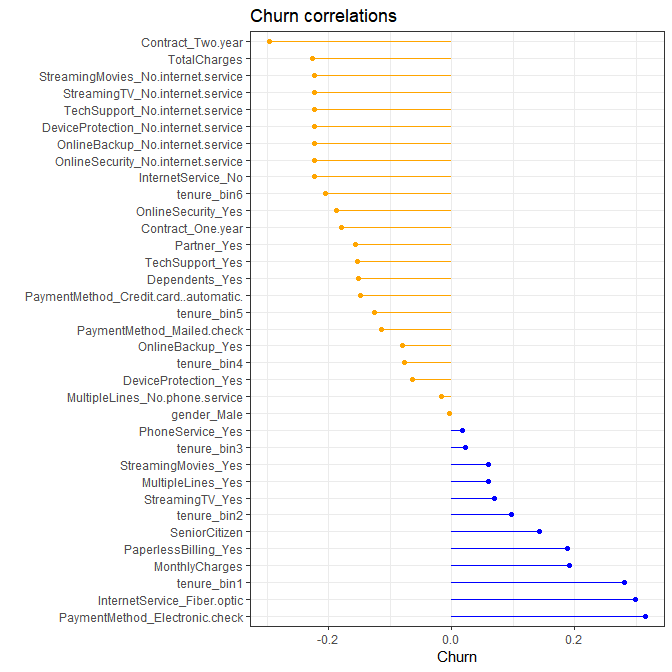## Install necessary packages

pkgs <- c("keras", "lime", "rsample", "recipes", "yardstick", "corrr")
install.packages(pkgs)

## tidyverse

http://tidyverse.org/

The tidyverse packages provide an easy way to import, tidy, transform and visualize the data. Some of it’s component R packages are:

• dplyr
• tidyr
• readr
• ggplot2
Parsed with column specification:
cols(
.default = col_character(),
SeniorCitizen = [32mcol_double()[39m,
tenure = [32mcol_double()[39m,
MonthlyCharges = [32mcol_double()[39m,
TotalCharges = [32mcol_double()[39m
)
See spec(...) for full column specifications.
glimpse(churn_data_raw)
Observations: 7,043
Variables: 21
$customerID [3m[38;5;246m<chr>[39m[23m "7590-VHVEG", "5575-GNVDE", "3668-QPYBK", "7795-CFOCW", "9237-H...$ gender           [3m[38;5;246m<chr>[39m[23m "Female", "Male", "Male", "Male", "Female", "Female", "Male", "...
$SeniorCitizen [3m[38;5;246m<dbl>[39m[23m 0, 0, 0, 0, 0, 0, 0, 0, 0, 0, 0, 0, 0, 0, 0, 0, 0, 0, 0, 0, 1, ...$ Partner          [3m[38;5;246m<chr>[39m[23m "Yes", "No", "No", "No", "No", "No", "No", "No", "Yes", "No", "...
$Dependents [3m[38;5;246m<chr>[39m[23m "No", "No", "No", "No", "No", "No", "Yes", "No", "No", "Yes", "...$ tenure           [3m[38;5;246m<dbl>[39m[23m 1, 34, 2, 45, 2, 8, 22, 10, 28, 62, 13, 16, 58, 49, 25, 69, 52,...
$PhoneService [3m[38;5;246m<chr>[39m[23m "No", "Yes", "Yes", "No", "Yes", "Yes", "Yes", "No", "Yes", "Ye...$ MultipleLines    [3m[38;5;246m<chr>[39m[23m "No phone service", "No", "No", "No phone service", "No", "Yes"...
$InternetService [3m[38;5;246m<chr>[39m[23m "DSL", "DSL", "DSL", "DSL", "Fiber optic", "Fiber optic", "Fibe...$ OnlineSecurity   [3m[38;5;246m<chr>[39m[23m "No", "Yes", "Yes", "Yes", "No", "No", "No", "Yes", "No", "Yes"...
$OnlineBackup [3m[38;5;246m<chr>[39m[23m "Yes", "No", "Yes", "No", "No", "No", "Yes", "No", "No", "Yes",...$ DeviceProtection [3m[38;5;246m<chr>[39m[23m "No", "Yes", "No", "Yes", "No", "Yes", "No", "No", "Yes", "No",...
$TechSupport [3m[38;5;246m<chr>[39m[23m "No", "No", "No", "Yes", "No", "No", "No", "No", "Yes", "No", "...$ StreamingTV      [3m[38;5;246m<chr>[39m[23m "No", "No", "No", "No", "No", "Yes", "Yes", "No", "Yes", "No", ...
$StreamingMovies [3m[38;5;246m<chr>[39m[23m "No", "No", "No", "No", "No", "Yes", "No", "No", "Yes", "No", "...$ Contract         [3m[38;5;246m<chr>[39m[23m "Month-to-month", "One year", "Month-to-month", "One year", "Mo...
$PaperlessBilling [3m[38;5;246m<chr>[39m[23m "Yes", "No", "Yes", "No", "Yes", "Yes", "Yes", "No", "Yes", "No...$ PaymentMethod    [3m[38;5;246m<chr>[39m[23m "Electronic check", "Mailed check", "Mailed check", "Bank trans...
$MonthlyCharges [3m[38;5;246m<dbl>[39m[23m 29.85, 56.95, 53.85, 42.30, 70.70, 99.65, 89.10, 29.75, 104.80,...$ TotalCharges     [3m[38;5;246m<dbl>[39m[23m 29.85, 1889.50, 108.15, 1840.75, 151.65, 820.50, 1949.40, 301.9...
$Churn [3m[38;5;246m<chr>[39m[23m "No", "No", "Yes", "No", "Yes", "Yes", "No", "No", "Yes", "No",... ## rsample https://tidymodels.github.io/rsample/ rsample contains a set of functions that can create different types of resamples and corresponding classes for their analysis. The goal is to have a modular set of methods that can be used across different R packages for: traditional resampling techniques for estimating the sampling distribution of a statistic and estimating model performance using a holdout set library(rsample) set.seed(100) train_test_split <- initial_split( churn_data_raw, prop = 0.3) train_tbl <- training(train_test_split) test_tbl <- testing(train_test_split) ## recipes https://tidymodels.github.io/recipes/ The recipes package is an alternative method for creating and preprocessing design matrices that can be used for modeling or visualization. library(recipes) rec_obj <- train_tbl %>% recipe(Churn ~ .) %>% step_rm(customerID) %>% step_naomit(all_outcomes(), all_predictors()) %>% step_discretize(tenure, options = list(cuts = 6)) %>% step_log(TotalCharges) %>% step_mutate(Churn = ifelse(Churn == "Yes", 1, 0)) %>% step_dummy(all_nominal(), -all_outcomes()) %>% step_center(all_predictors(), -all_outcomes()) %>% step_scale(all_predictors(), -all_outcomes()) %>% prep() summary(rec_obj) Save the recipe file into the Shiny folder for later use save(rec_obj, file = "../shiny-app/rec_obj.RData") Use juice() to extarct the results from the model preparation. The predictor and outcome data are processed separately. This is because how Keras expects these arguments to be used when fitting a model. x_train_tbl <- juice(rec_obj, all_predictors(), composition = "matrix") y_train_vec <- juice(rec_obj, all_outcomes()) %>% pull() The same is done with the testing data so that the two can be compared. baked_test <- bake(rec_obj, test_tbl) x_test_tbl <- baked_test %>% select(-Churn) %>% as.matrix() y_test_vec <- baked_test %>% select(Churn) %>% pull() ## Install Tensorflow & Keras https://tensorflow.rstudio.com/tensorflow/articles/installation.html https://tensorflow.rstudio.com/keras/#installation library(tensorflow) library(keras) #install_tensorflow() #install_keras() ### Create Neural Network model_keras <- keras_model_sequential() %>% layer_dense( units = 16, kernel_initializer = "uniform", activation = "relu", input_shape = ncol(x_train_tbl)) %>% layer_dropout(rate = 0.1) %>% layer_dense( units = 16, kernel_initializer = "uniform", activation = "relu") %>% layer_dropout(rate = 0.1) %>% layer_dense( units = 1, kernel_initializer = "uniform", activation = "sigmoid") %>% compile( optimizer = 'adam', loss = 'binary_crossentropy', metrics = c('accuracy') ) model_keras Model _____________________________________________________________________________________________ Layer (type) Output Shape Param # ============================================================================================= dense_1 (Dense) (None, 16) 576 _____________________________________________________________________________________________ dropout_1 (Dropout) (None, 16) 0 _____________________________________________________________________________________________ dense_2 (Dense) (None, 16) 272 _____________________________________________________________________________________________ dropout_2 (Dropout) (None, 16) 0 _____________________________________________________________________________________________ dense_3 (Dense) (None, 1) 17 ============================================================================================= Total params: 865 Trainable params: 865 Non-trainable params: 0 _____________________________________________________________________________________________ ### Fit model # Fit the keras model to the training data history <- fit( object = model_keras, x = x_train_tbl, y = y_train_vec, batch_size = 50, epochs = 35, validation_split = 0.30, verbose = 0 ) 2019-03-13 13:43:31.622924: I T:\src\github\tensorflow\tensorflow\core\platform\cpu_feature_guard.cc:141] Your CPU supports instructions that this TensorFlow binary was not compiled to use: AVX2 print(history) Trained on 1,478 samples, validated on 634 samples (batch_size=50, epochs=35) Final epoch (plot to see history): val_loss: 0.4457 val_acc: 0.7981 loss: 0.3909 acc: 0.816  ### Preview results theme_set(theme_bw()) # Plot the training/validation history of our Keras model plot(history)# Predicted Class yhat_keras_class_vec <- model_keras %>% predict_classes(x_test_tbl) %>% as.factor() %>% fct_recode(yes = "1", no = "0") # Predicted Class Probability yhat_keras_prob_vec <- model_keras %>% predict_proba(x_test_tbl) %>% as.vector() test_truth <- y_test_vec %>% as.factor() %>% fct_recode(yes = "1", no = "0") # Format test data and predictions for yardstick metrics estimates_keras_tbl <- tibble( truth = test_truth, estimate = yhat_keras_class_vec, class_prob = yhat_keras_prob_vec ) estimates_keras_tbl ## yardstick https://tidymodels.github.io/yardstick/ yardstick is a package to estimate how well models are working using tidy data principals. library(yardstick) options(yardstick.event_first = FALSE) # Confusion Table estimates_keras_tbl %>% conf_mat(truth, estimate)  Truth Prediction no yes no 3247 564 yes 378 731 # Accuracy estimates_keras_tbl %>% metrics(truth, estimate)  # AUC estimates_keras_tbl %>% roc_auc(truth, class_prob)  # Precision estimates_keras_tbl %>% precision(truth, estimate) %>% bind_rows( estimates_keras_tbl %>% recall(truth, estimate) )   # F1-Statistic estimates_keras_tbl %>% f_meas(truth, estimate, beta = 1) ## lime https://github.com/thomasp85/lime The purpose of lime is to explain the predictions of black box classifiers. What this means is that for any given prediction and any given classifier it is able to determine a small set of features in the original data that has driven the outcome of the prediction. library(lime) model_type.keras.engine.sequential.Sequential <- function(x, ...) { "classification" } # Setup lime::predict_model() function for keras predict_model.keras.engine.sequential.Sequential <- function(x, newdata, type, ...) { pred <- predict_proba(object = x, x = as.matrix(newdata)) data.frame(Yes = pred, No = 1 - pred) } # Test our predict_model() function model_keras %>% predict_model(x_test_tbl, "raw") %>% as_tibble() library(lime) # Run lime() on training set explainer <- x_train_tbl %>% as_tibble() %>% lime(model_keras, bin_continuous = FALSE) # Run explain() on explainer explanation <- x_train_tbl %>% as.data.frame() %>% head(40) %>% lime::explain( explainer = explainer, n_labels = 1, n_features = 4, kernel_width = 0.5 ) plot_explanations(explanation) + labs(title = "LIME Feature Importance Heatmap", subtitle = "Hold Out (Test) Set, First 40 Cases Shown")## corrr https://github.com/drsimonj/corrr corrr is a package for exploring correlations in R. It focuses on creating and working with data frames of correlations (instead of matrices) that can be easily explored via corrr functions or by leveraging tools like those in the tidyverse. library(corrr) corrr_analysis <- x_train_tbl %>% as_tibble() %>% mutate(Churn = y_train_vec) %>% correlate() %>% focus(Churn) %>% rename(feature = rowname) %>% arrange(abs(Churn)) %>% mutate(feature = as_factor(feature))   Correlation method: 'pearson' Missing treated using: 'pairwise.complete.obs' corrr_analysis over <- corrr_analysis %>% filter(Churn > 0) under <- corrr_analysis %>% filter(Churn < 0) corrr_analysis %>% ggplot(aes(x = Churn, y = fct_reorder(feature, desc(Churn)))) + geom_point() + geom_segment(aes(xend = 0, yend = feature), data = under, color = "orange") + geom_point(data = under, color = "orange") + geom_segment(aes(xend = 0, yend = feature), data = over, color = "blue") + geom_point(data = over, color = "blue") + labs(title = "Churn correlations", y = "")NA ## Some more exploration churn_data_raw %>% group_by(Contract, Churn) %>% tally() %>% spread(Churn, n) churn_data_raw %>% group_by(InternetService, Churn) %>% tally() %>% spread(Churn, n) ## Deploying the model ### Save the Keras model export_savedmodel(model_keras, "tfmodel") ## Deploy to RStudio Connect library(rsconnect) deployTFModel( "tfmodel", server = "colorado.rstudio.com", account = rstudioapi::askForPassword("Enter Connect Username:") ) ### Test the deployed model library(httr) baked_numeric <- x_test_tbl %>% as_tibble() %>% head(4) %>% transpose() %>% map(as.numeric) body <- list(instances = list(baked_numeric)) r <- POST("https://colorado.rstudio.com/rsc/content/2230/serving_default/predict", body = body, encode = "json") jsonlite::fromJSON(content(r))$predictions[, , 1]
 0.5589350 0.2727856 0.5589350 0.5589350